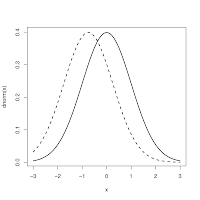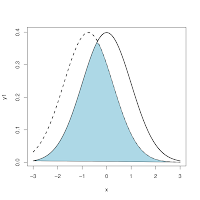## Wednesday, 19 September 2007

### Area under two curves in [R]As it's Talk Like a Pirate Day, it seemed a good time to share a recent insight from working with the statistical system R. Why the pirate link? R, Jimlad...

Anyway, I recently had two overlapping curves, like in the first diagram, and wanted a way of finding the area that fell under the two without getting too complex, as one must normally do. I've a horrible feeling I'm about to say something really obvious as though it's profound (not for the first time), but having meddled for ages trying to find a way of doing it, it was something of a revelation to me suddenly to realize that for practical purposes (i.e., for simulations and graphs) you can find this area simply by taking the minimum y-value for each value of x. The lowest curve at any point is the upper-bound on the common area, so working across the x values taking the minimum is a really simple way to extract the shared region. Let's first create some sample data - these are the two curves in the diagram...

`x <- seq(-3,3,0.1) #create a sequence of x valuesy1 <- dnorm(x) #create the first curvey2 <- dnorm(x + 0.75) # create the second curveplot(x,y1) # graph the first curvelines(x,y2,lty=2) # overlay the second`

Then find the minimum at each point...

`common <- array() #create a new array to hold the common areafor (i in 1:length(x)) { #for each value of x...common[i] <- min(y1[i],y2[i]) #...take the lowest y across the two curves}`This gives you the variable called 'common' which represents this shared area, and you can do all sorts of things with this, such as plotting a coloured region as I have in my second diagram.

`polygon(c(min(x), x, max(x)), c(0, common, 0), col="lightblue")`

As I say, this might be obvious to most of you, but it was a useful thing for me to work out so I hope it may help others. R, Jimlad.

#### 8 comments:

nico said...

It was in fact really obvious... after I read it here! Thanks!

nico

Ian Walker said...

I'm really pleased someone found this useful!Anonymous said...

Useful to me as well

merci!

kanch said...

just what i was looking for... Thanks a lot :)

Unknown said...

Thanks! That was really useful. Also, if I want to find the area of the region shared, how would I go about doing that?

Ian Walker said...

Okay, this COULD be wrong, as it's just off the top of my head, but you can do:

sum(y1)

to get the area under one curve (in arbitrary units), and sum(y2) to get the area under the other (they should be approximately equal, with any differences due to rounding errors etc.). You can also use

sum(common)

to get the shared area. So if you do

sum(common) / sum(y1)

you'll get the size of the common area in standardized units (i.e., as a proportion of each original curve). I think. Hope that helps.

Unknown said...

In simplicity lies genius.
Beautiful man, just what I needed.

You can then plug "common" into sintegral() in Bolstad2 package and get area between the curves.

Unknown said...

In simplicity lies genius.
Awesome man, just what I was looking for.
Then you can dump "common" into sintegral() in Bolstad2 library and get area between the curves. Nice.